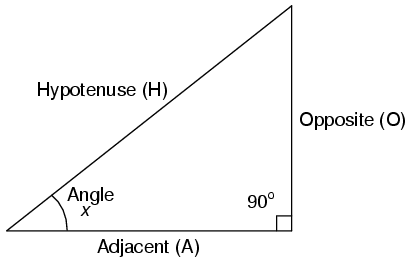# Right Triangle TrigonometryA right triangle is defined as having one angle precisely equal to 90o (a right angle).

### Trigonometric Identities

H is the Hypotenuse, always being opposite the right angle. Relative to angle x, O is the Opposite and A is the Adjacent.

"Arc" functions such as "arcsin", "arccos", and "arctan" are the complements of normal trigonometric functions. These functions return an angle for a ratio input. For example, if the tangent of 45o is equal to 1, then the "arctangent" (arctan) of 1 is 45o. "Arc" functions are useful for finding angles in a right triangle if the side lengths are known.

### The Pythagorean Theorem

##### Lessons In Electric Circuits copyright (C) 2000-2020 Tony R. Kuphaldt, under the terms and conditions of the CC BY License.

See the Design Science License (Appendix 3) for details regarding copying and distribution.

Revised July 25, 2007Swipe left and right to change pages.